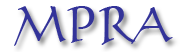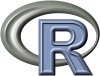Home

Sup-tests for linearity in a general nonlinear AR(1) model

Christian Francq, Lajos Horvath and Jean-Michel Zakoïan
Keywords: Diagnostic checking; Exponential autoregressive (EXPAR) model; Lagrange Multiplier (LM) tests; Least Squares Estimator; Likelihood Ratio (LR); Non Linear models; Supremum Tests; Smooth Transition Autoregressive (STAR); Threshold AR (TAR); Wald test
Abstract: We consider linearity testing in a general class of nonlinear time series model of order 1, involving a nonnegative nuisance parameter which (i) is not identified under the null hypothesis and (ii) gives the linear model when equal to zero. This paper studies the asymptotic distribution of the Likelihood Ratio test and asymptotically equivalent supremum tests. The asymptotic distribution is described as a functional of chi-square processes and is obtained without imposing a positive lower bound for the nuisance parameter. The finite sample properties of the sup-tests are studied by simulations.MPRA working paper
R code used to obtainFigure 1